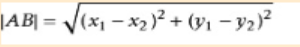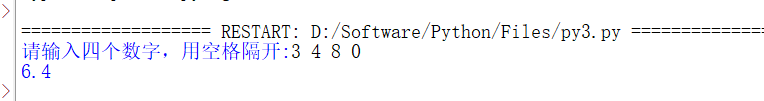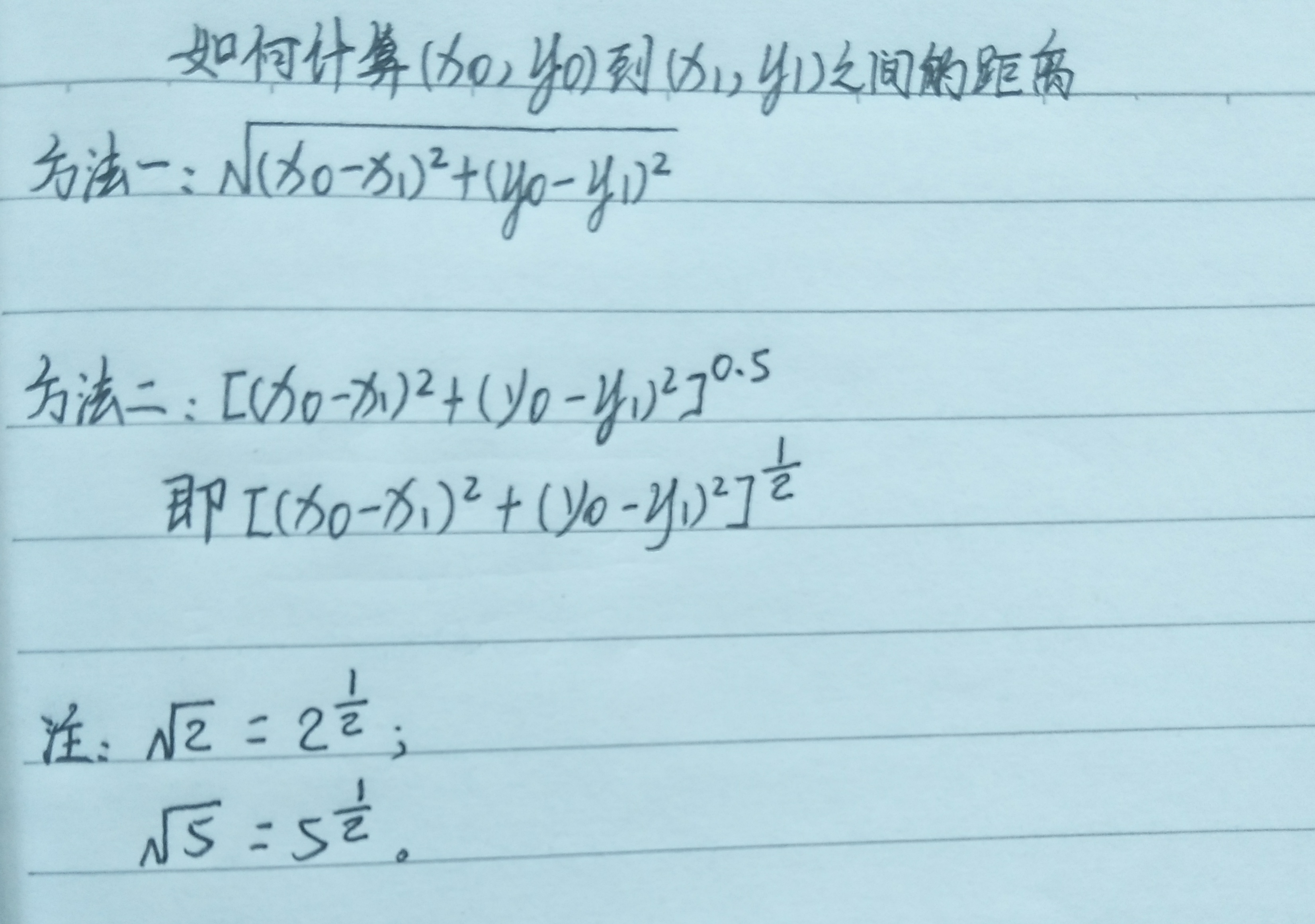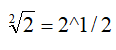• python 计算两点之间距离
2022-04-04 12:20:12

已知两个点的经度和纬度，计算两个点之间的距离

import pyproj

lat1,long1 = (37.8101,-122.4105)
lat2,long2 = (37.8024,-122.4058)

geod = pyproj.Geod(ellps="WGS84")

angle1,angle2, distance = geod.inv(long1,lat1,long2,lat2)

print(format(distance))


该例子来自《Python Geospatial Development》一书，此处仅作为学习笔记。

更多相关内容
• 今天小编就为大家分享一篇Python两点之间的直线距离(2种实现方法)，具有很好的参考价值，希望对大家有所帮助。一起跟随小编过来看看吧
• 今天小编就为大家分享一篇python计算两个地址之间距离方法，具有很好的参考价值，希望对大家有所帮助。一起跟随小编过来看看吧
• 计算两点(x1,y1),(x2,y2)之间距离，输出并保留一位小数 两点之间距离的数学公式： txt=input("请输入四个数字，空格隔开:") ls=txt.split(" ") x1=eval(ls) y1=eval(ls) x2=eval(ls) y2=eval(ls) r...

从键盘输入4个数字，采用空格分隔，对应变量为x1,y1,x2,y2。计算两点(x1,y1),(x2,y2)之间的距离，输出并保留一位小数

两点之间距离的数学公式：txt=input("请输入四个数字，用空格隔开:")
ls=txt.split(" ")
x1=eval(ls)
y1=eval(ls)
x2=eval(ls)
y2=eval(ls)
r=pow(pow(x1-x2,2)+pow(y1-y2,2),0.5)
print("{:.1f}".format(r))展开全文开发语言 后端
• 如下所示： distances = np.sqrt(np.sum(np.asarray(airportPosition - x_vals)**2, axis=1)) ... 您可能感兴趣的文章:python计算两个地址之间距离方法Python实现的计算马氏距离算法示例Python实现计
• ## 用Python如何计算两点间距离

万次阅读 多人点赞 2020-03-02 17:27:36
用Python如何计算两点间距离？ 第一步，我们先来看一下两点距离公式。 两点间距离公式： ？？？这是个啥？ 是不是方法一还能看懂，方法二却一脸懵逼？ 请仔细看注释， 也就是说，根号2还可以写成2的...

# 用Python如何计算两点间距离？

## 第一步，我们先来看一下两点间的距离公式。

### 两点间距离公式：## ？？？这是个啥？是不是方法一还能看懂，方法二却一脸懵逼？

## 请仔细看注释，### 也就是说，根号2还可以写成2的0.5(也就是1/2)次方

这个是高中学过的知识，不知道你有没有想起来，哈哈......

## 第二步，我们需要学习一下pow()这个函数

### pow(a,b)是计算a的b次方的一个函数

例如：pow(2,3)为2的3次方，为8

## 第三步，split()函数

### split()函数是将数据按指定格式分割开，默认为空格

例1：

a = "0 1 3 5"

print(a.slpit())

将a中的数据以空格的分割开，并返回一个['0', '1', '3', '5']形式的列表

例2：

a = 0,1,3,5

print(s.split(","))

将a中的数据以逗号分割开，并返回一个['0', '1', '3', '5']形式的列表

## 第四步 format()格式化

### format()格式化与%s, %d, %f有异曲同工之效，都是先为数据占一个位，而后补充数据

例1：

weekday = "星期一"

print('今天是%s' % weekday)

显示为：今天是星期一

例2：

weekday = "星期一"

print('今天是{}'.format(weekday))

### 第五步，我们看一下具体的代码~

从键盘上输入4个数字，各数字采用空格分隔，对应变量为x0, y0, x1, y1。计算两点(x0, y0)和(x1, y1)之间的距离。在屏幕上输出这个距离，保留2位小数。例如，键盘输入0 1 3 5，屏幕输出5.00

  #输入数据
ntxt=input('请输入4个数字(空格分隔):')
#利用split()函数，通过空格将数据分隔开并赋值给变量nls
nls=ntxt.split()
#利用eval()函数将字符串类型转化为数据类型；
#nls，将nls中的第零个数据赋值给变量x0
x0=eval(nls)
#nls，将nls中的第一个数据赋值给变量y0
y0=eval(nls)
#nls，将nls中的第二个数据赋值给变量x1
x1=eval(nls)
#nls，将nls中的第三个数据赋值给变量y1
y1=eval(nls)
#利用pow()函数计算两点间距离
r=pow(pow(x1-x0,2)+pow(y1-y0,2),0.5)
#打印数据；其中2f表示保留两位小数
print('{:.2f}'.format(r))

注：print('{:.2f}'.format(r))的意思是：用{:.2f}先占一个位置; .2f表示保留两位小数。

展开全文• My question is there a function or library for python? 解决方案from math import * def greatCircleDistance((lat1, lon1), (lat2, lon2)): def haversin(x): return sin(x/2)**2 return 2 * asin(sqrt( ...I get longitude and latitude using gmail geocode function. Now i need to calculate distance between 2 points. I found Haversine formula and it works well, but then in google js api i found method computeDistanceBetween(lat,lng) in the Geometry Library. My question is there a function or library for python?

解决方案from math import *

def greatCircleDistance((lat1, lon1), (lat2, lon2)):

def haversin(x):

return sin(x/2)**2

return 2 * asin(sqrt(

haversin(lat2-lat1) +

cos(lat1) * cos(lat2) * haversin(lon2-lon1)))

This returns the angular distance between the points on a sphere. To get a distance in length (kilometers), multiply the result with the Earth radius.

But you could have it looked up in a formula collection as well, hence the bunch of downvotes ;-)

展开全文• 计算两点之间距离日常旁白：本人是一枚生物学的学生，由于对生物信息学特别感兴趣，于是想自学生物信息学（新手莫怪）。了解到生物信息学要有编程基础，尤其是要会一门编程语言，例如：R语言、Python、Perl等，...
• 计算是在您提取的二维灰色矩阵上进行的。我已经为输入到cluster的矩阵的感兴趣的值指定了0和1，在本例中是KMeans，尽管可以根据需要其他模型替换它。import cv2import numpy as npfilename = 'Dog.jpg'img = cv2....
• #coding:UTF-8 """ Python implementation of Haversine formula Copyright (C) <2009> Bartek Górny This program is free software: you can redistribute it and/or modify it under the terms of the GNU ...
• python模块：base64 base64模块是用来作base64编码解码的,在电子邮件中常见.它可以把不能作为文本显示的二进制数据编码为可显示的文本信息,编码后文本大小增加1/3.常用方法有: b64encode & b ... DSP using MATLAB ...
• "haversine公式计算球面两点间距离。" # 经纬度转换成弧度 lat0 = radians(lat0) lat1 = radians(lat1) lng0 = radians(lng0) lng1 = radians(lng1) dlng = fabs(lng0 - lng1) dlat = fabs(lat0 - lat1...
• 角度检测 Python-OpenCV应用计算两点之间的角度 如果您打开该程序的LIVE版本，它将打开设置，在此处应管理所需颜色的HSV范围 如果您打开非实时版本，则应选择想要使用的图片
• from math import radians, cos, sin, asin, sqrt   def haversine(lon1, lat1, lon2, lat2): # 经度1，纬度1，经度2，纬度2   """  Calculate the great circle distance between two points  ...
• 我需要创建一个列表,找到最接近的,然后打印出来.如何比较列表中的每个？没有任何需要绘制或任何东西,只是比较,找到列表中最接近的个.import math # 'math' needed for 'sqrt'# Distance functiondef ...
• 比如 A 点经纬度（30.553949，114.357399），B点经纬度（129.1344，25.5465），求 AB 两点之间距离。 已知地球上任意两点（lng1，lat1），（lng2， lat2）的经纬度坐标，求两点间距离可以利用 haversine 公式： ...haversine geopy
• 今天小编就为大家分享一篇python 计算方位角实例(根据两点的坐标计算)，具有很好的参考价值，希望对大家有所帮助。一起跟随小编过来看看吧
• 今天小编就为大家分享一篇python实现个经纬度点之间距离和方位角的方法，具有很好的参考价值，希望对大家有所帮助。一起跟随小编过来看看吧
• 类似的需求应该比较多，这里的核心是关于个坐标点之间距离计算，之前没用过这个东西，刚好今天有个这样的需求，记录下。 import requests import psycopg2 import pandas as pd from math import radians, ...
• 计算Python Numpy向量之间的欧氏距离，已知vec1和vec2是两个...# 补充知识：Python计算两个数据点之间的欧式距离，一个到数据集中其他距离之和 # 如下所示： # 计算数两个数据点之间的欧式距离 import npytorch
• 匿名用户1级2018-05-29 回答#导入math包importmath#定义的函数classPoint:x=0y=0z=0def__init__(self,x,y,z):self.x=xself.y=yself.z=zdefgetx(self):returnself.xdefgety(self):returnself.ydefgetz(self)......